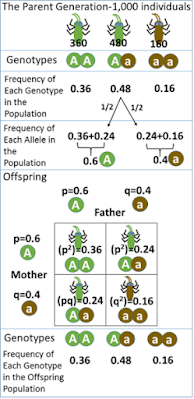## Friday, March 25, 2022

### Genetic Equilibrium and the Hardy Weinberg Principle

Biology Index

Where are we going with this? The information on this page should increase understanding related to this standard:  Identify chronological patterns of change and communicate that biological evolution is supported by multiple lines of empirical evidence that identify similarities inherited from a common ancestor (homologies).

Article includes ideas, images, and content from Troy Smigielski (2022-01)

Genetic Equilibrium and the Hardy Weinberg Principle
(This guy sounds like some stand-up comedian. Just saying…)

Genetic equilibrium is the situation in which allele frequencies remain constant through time. REMEMBER: Allele frequencies are the frequencies of alleles in a population, often expressed as a fraction, percentage, or decimal.

So… In a population of 100 turtles if…

64 turtles are “BB”
12 turtles are “Bb”
24 turtles are “bb”

Then we can figure out the frequency of B and b (which is the allele frequency). How many B's are there? How many b's are there?

Math… then, we can say that the B allele frequency is 70% and the b allele frequency is 30%.

The Hardy-Weinberg Principle states that allele frequencies in a population will remain constant under 5 conditions:

1. No natural selection occurs in a population.
2. No migration into or out of a population (no gene flow).
3. No mutations happen in the gene pool of a population.
4. Random mating throughout the population.
5. Large populations of organisms to keep frequencies constant.
That is to say that…

…in a large, random-mating population that is not affected by the evolutionary processes of mutation, migration, or natural selection, allele frequencies (and genotype frequencies) will remain constant throughout time.

Psst… THIS IS NOT COMMON IN REAL LIFE.Source, 2022-03
This can be thought of as all of the parts adding up to one. All of the fractions of alleles will add up to one.  Sometimes it is shown like this:

Hardy-Weinberg Equations for Genetic Equilibrium

Where "frequency" is expressed as a decimal representing how many there are compared to the total number and where…

p = frequency of dominant allele (A)
q = frequency of recessive allele (a)

then…
p + q = 1

and further…

p2 + 2pq + q2 = 1

will also be true so that…

2pq = frequency of heterozygous organisms (Aa)
p2 = frequency of homozygous dominant organisms (AA)
q2 = frequency of homozygous recessive organisms (aa)

______________________
Example:

You have sampled a population in which you know that the percentage of the homozygous recessive genotype (aa) is 36%. Find the allele frequency and the genotype frequency for the population.

Given that 36% are homozygous recessive…
That means that

q2 = 36% = .36

Which means that q = .6

We can then solve for p…

p + q = 1
p + .6 = 1
p = 1 = .6
p = .4

So… if p = .4 then…

p= .16

Now, we can find the heterozygous frequency; it is equal to 2pq

2pq = 2 • .4 • .6
2pq = .48

We can check that…

p2 + 2pq + q2 = 1
.16 +  .48 + 36 = 1

Now, we can put that into words…

The frequency of the "a" allele is .6.
The frequency of the "A" allele  .4.

The frequency of the genotype "aa" is .36. <-- this was given
The frequencies of the genotype "AA" .16.
The frequencies of the genotype "Aa" is .48.

______________________

Why Does This Matter?
• This principle provides a baseline to compare actual populations to.

•  Step 1: Find allele frequencies in year 1

•  Step 2: Find allele frequencies in year 5

• If a population is in genetic equilibrium, then the allele frequencies from Year 1 would equal Year 5. If Year 5 shows different allele frequencies, then we know that genetic drift is happening, and we can find out how much a population has changed.

• With this information, we can see how quickly a population is changing.

In essence, this process allows a means to demonstrate genetic drift within a given population, which indicates that something is changing. Microevolution can be, therefore, measured and described in terms of changes in the present allele frequencies.